# Shortcut keys for inserting Greek symbols into the equation

Word
In Word 2007 equation many symbols can be inserted using a \+name of the symbol: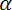\alpha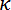\kappa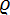\varrho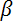\beta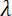\lambda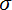\sigma\chi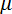\mu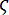\varsigma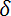\delta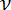\nu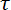\tau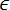\epsilon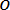\o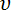\upsilon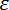\varepsilon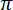\pi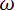\omega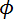\phi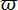\varpi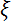\xi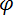\varphi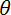\theta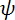\psi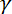\gamma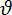\vartheta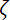\zeta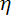\eta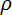\rho

See Shortcut keys for inserting symbols and templates into the equation to find other frequently used symbols.

To insert a capital letter of the Greek alphabet, simply enter \+Name of the symbol starting with a capital letter: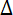\Delta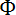\Phi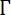\Gamma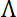\Lambda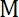\Mu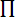\Pi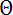\Theta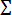\Sigma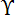\Upsilon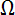\Omega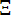\Hi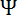\Psi

How to insert other symbols and templates in an equation, see Shortcut keys for inserting symbols and templates into the equation.

How to use all these symbols outside the equation, select the option Use Math AutoCorrect rules outside of math regions in the Word Options. How to do it, see Choosing Math AutoCorrect Options.

If you have any questions or suggestions, please feel free to ask OfficeToolTips team.